### Home > MC1 > Chapter 6 > Lesson 6.3.1 > Problem6-121

6-121.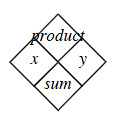6-121. Copy and complete each of the Diamond Problems below. The pattern used in the Diamond Problems is shown at right. Homework Help ✎

 a.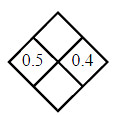b.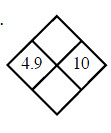c.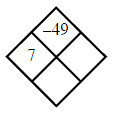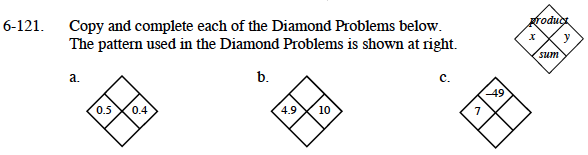Begin by multiplying x and y to fill the product box.
0.5 · 0.4 = 0.2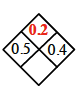Then add x and y together to fill the sum box.
0.5 + 0.4 = 0.9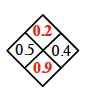Follow the steps as outlined in part (a).

You will know you are right when the product is equal to −49.

Add x and y to complete the sum box.